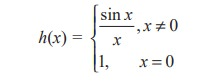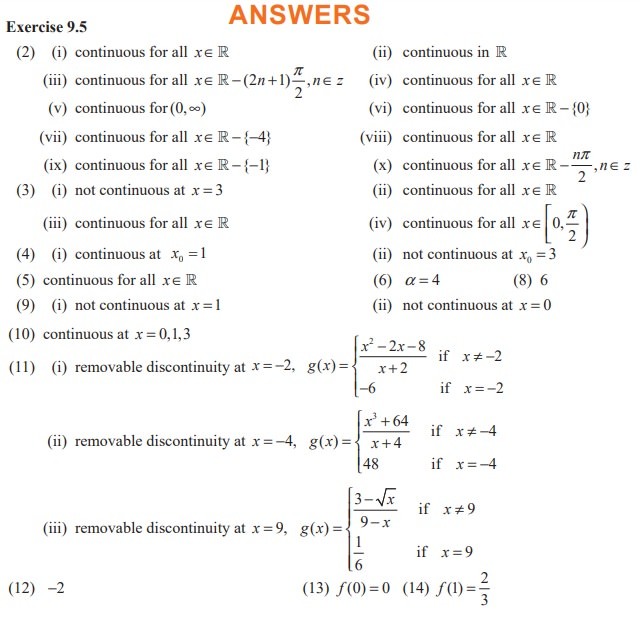Home | | Maths 11th std | Removable and Jump Discontinuities - Differential Calculus

# Removable and Jump Discontinuities - Differential Calculus

Let us look at the following functions :

Removable and Jump Discontinuities

Let us look at the following functions :

(i) f(x) = sinx / x

(ii) g ( x ) = C ( x), where C(x) is as defined in Example 9.37.

The function f(x) is defined at all points of the real line except x = 0. That is, f(0) is undefined, butexists. If we redefine the function f(x) ash is defined at all points of the real line including x = 0. Moreover, h is continuous at x =0 sinceNote that h(x) = f(x) for all x 0. Even though the original function f(x) fails to be continuous at x = 0, the redefined function became continuous at 0. That is, we could remove the discontinuity by redefining the function. Such discontinuous points are called removable discontinuities. This example leads us to have the following.

Definition 9.10

A function f defined on an interval I ⊆ R is said to have removable discontinuity at x0 I  if there is a function h:I→R such that

h(x) =Note that for removable discontinuity, limx x0 f (x) must exist.

Now if we examine the function g(x) = C(x) (see Example 9.38) , eventhough it is defined at all points of  [0, ∞), lim x→100 g (x) does not exist and it has a jump of height lim x →100+ g (x ) − lim x→100− g (x) = 16 − 14 = 2, which is finite. Since lim x→100 g (x) does not exist, it is not continuous at x = 100. Such discontinuities are called jump discontinuities. Thus we have the following :

### Definition 9.11

Let f be a function defined on an interval I R. Then f is said to have jump discontinuity at a point x0 I if f is defined at x0,EXERCISE 9.5Tags : Definition, Solved Example Problems, Exercise | Mathematics , 11th Mathematics : UNIT 9 : Differential Calculus Limits and Continuity
Study Material, Lecturing Notes, Assignment, Reference, Wiki description explanation, brief detail
11th Mathematics : UNIT 9 : Differential Calculus Limits and Continuity : Removable and Jump Discontinuities - Differential Calculus | Definition, Solved Example Problems, Exercise | Mathematics